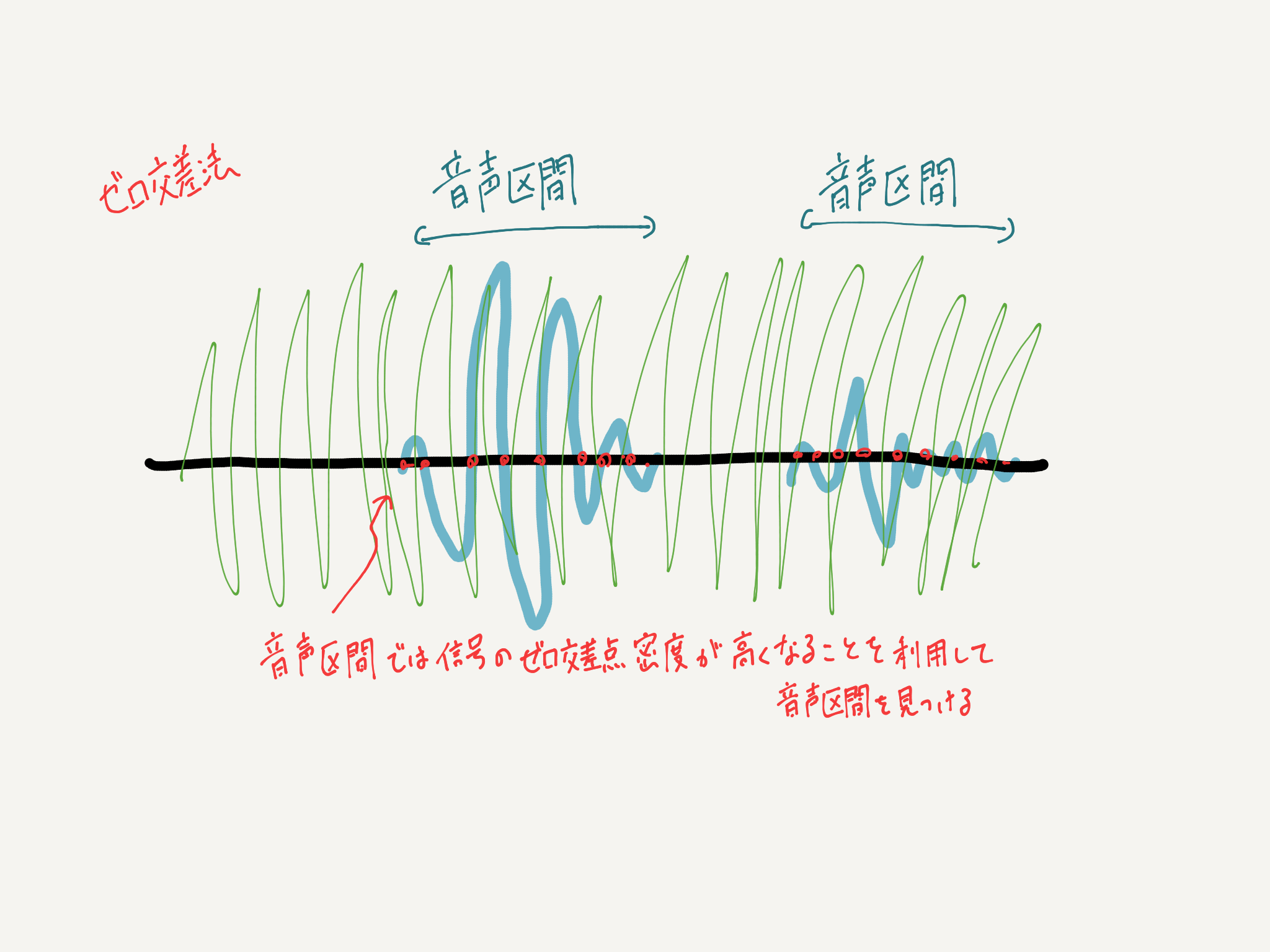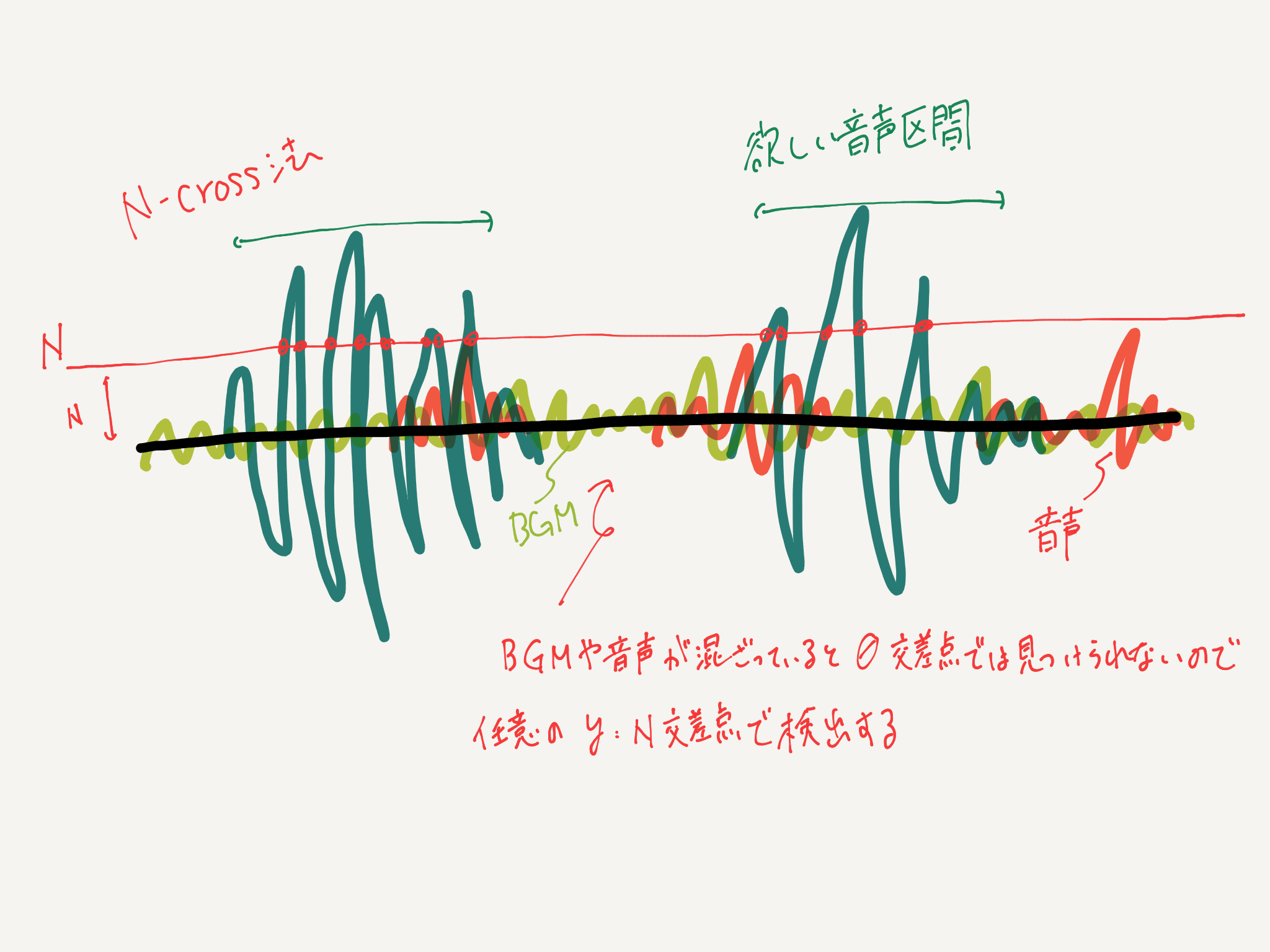# N-cross method

When Blind Separation (BASS) for voice was applied by ILRMA, the voice became a suppressed separation instead of a complete separation (zeroing the voice of the speaker who was not the target). It is a method devised so that even such voice can be {VAD}. The name N-cross method is a name I gave it appropriately, so I don't think you can find any references by searching.

# Overview

There is a method called zero crossing method used for voice activity detection (VAD), but since this is weak against noise resistance, it is generally a frame based on a Gaussian mixture model (GMM). Section detection is performed based on unit voice / non-voice identification. However, with the sound source separated by ILRMA this time, although it is possible to suppress the sound pressure level for unintended voice, it cannot be completely reduced to zero, so even the voice of the part that is not necessary by the GMM method is treated as the utterance section. It will end up. Therefore, we implemented a method that can detect the target voice even if there is voice in the background. This method will be called the "N-cross method" this time. I think that this method can be used not only for this method, but also for situations such as business negotiations at a cafe where external audio is likely to be mixed in, or when BGM is included in the sound source.# theory

The theory is simple. In the zero intersection method, the section where the frequency of intersection at the line of Y = 0 is high with respect to the voice waveform is detected as the utterance section, but with this method, even a small voice is detected, so N- The cross method uses an intersection with an arbitrary value of Y = N. We will call this N value * N-cross Line * or * Sensitivity *.# Implementation

Implemented in Python. Please run it with Colab or Jupyter.

In the following ``Ncross ()` `, if you input a monaural sound source` `data```, it will be less than or equal to` `N``` (= 0 to 1) when the maximum sound pressure of the sound source is 1. If the sound pressure of is not considered as a VAD target, and the voice data is divided into` `hop_length``` and detected as the target speech section, it is set to "1", otherwise it is set to "0". Returns a timeline list. In addition, the parameter `` `m``` gives the area value that considers the target interval as a voice interval. The smaller it is, the more severe it becomes, and the larger it is, the more noise it picks up.

And in the ` kukan ()` `` written below, start talking from the actual voice using the time axis list `` `N_cross_list` obtained by ``Ncross ()``. Returns a list that stores the time index of and the time index of the end of the story. Regarding the parameters, in ` N_cross_list`, the utterance section is given by "1" and the silence section is given by "0", so if this value is given by ``num```, any interval You can get the time index of. Next is` `M```, but in conversation, I think you can take a break even while you are talking. In the utterance with this silence, it is a value that determines how many seconds the silence is considered as a continuous conversation. Since `` `M = 200` is set this time, the sampling frequency is 44000 in the actual time, so `` `M * hop_length / SampleRate = 2.32 ...` ``, which is about 2 seconds. If there is a degree of silence, it is regarded as a break in the speech section. Also, although not included in the input parameters, `` `chousei` is a parameter that gives a margin to the detected utterance section. Since ``chousei = 30``` is set here,` `chousei * hop_length / SampleRate = 0.348 ...` `` seconds is considered to be the utterance section. The reason why I do this is because the fricatives and plosives at the beginning of conversation are often not detected as voice.

#### `Ncross.py`

``````
#N-Cross method
#0 in the silent section,Returns a timeline list of 1 in the voiced interval

def Ncross(data,N,m,hop_length):
"""
N-Cross method
1 in the silent section,Returns a timeline list of 0 in the voiced interval
'''
data : Sound
N :Area value(0~1)
m :Threshold in the zero-cross method
hop_length :Number of cuts in the frame
"""

y=data/np.max(np.abs(data))
#Number of cuts in the frame
nms = ((y.shape)//hop_length)+1

#Zero padding so that the beginning and end can be cut out with a frame
y_bf = np.zeros(hop_length*2)
y_af = np.zeros(hop_length*2)
y_concat = np.concatenate([y_bf, y, y_af])

zero_cross_list = []
for j in range(nms):
zero_cross = 0
#Cut out by frame
y_this = y_concat[j*512:j*512+2048]
for i in range(y_this.shape-1):
#Condition if positive or negative changes
if (np.sign(y_this[i]-N) - np.sign(y_this[i+1]-N))!=0:
zero_cross += 1
zero_cross_list.append(zero_cross)

#Normalized to a maximum of 1
zero_cross_list = np.array(zero_cross_list)/max(zero_cross_list)

#Threshold is 0.Set to 4 (heuristic, but ...)
zero_cross_list = (zero_cross_list<m)*1
return zero_cross_list

hop_length = 2**9
cross_list = []
for i in range(N_person):
N_cross_list= Ncross(sep[:, -(i+1)],0.3,0.2,hop_length)
cross_list.append(N_cross_list)
N_cross_list1 = cross_list
N_cross_list2 = cross_list
for i in range(N_person):
plt.plot(sep[:, -(i+1)])
plt.show()
``````

#### `separation.py`

``````
num = 2
M = 200
chousei = 30
def kukan(N_cross_list,num,M):
A = N_cross_list
A_index = np.where(A == num)
startlist = [A_index]
endlist = []
for i in range(len(A_index)-1):
dif = A_index[i+1]-A_index[i]
if dif > M:
endlist.append(A_index[i])
startlist.append(A_index[i+1])
endlist.append(A_index[-1])
return startlist,endlist
for i in range(N_person):
startpoint,endpoint = kukan(cross_list[i],num=0,M=M)
print("start point",startpoint)
print("end point",endpoint)
for n in range(len(startpoint)):
target = sep[:, -(i+1)]
audio=target[hop_length*(startpoint[n]-chousei):hop_length*(endpoint[n]+chousei)]
display(Audio(audio, rate=RATE))
``````